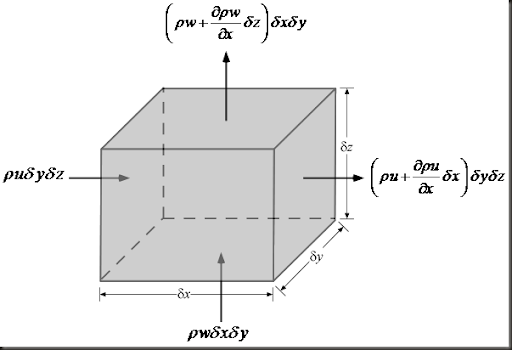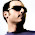## Saturday, February 21, 2009

### 7. Derivation of the Continuity Equation in Cartesian Coordinates

[Previous Article: How Euler Derived the Continuity Equation]
The continuity equation is an expression of a fundamental conservation principle, namely, that of mass conservation. It is a statement that fluid mass is conserved: all fluid particles that flow into any fluid region must flow out. To obtain this equation, we consider a cubical control volume inside a fluid. Mass conservation requires that the the net flow through the control volume is zero. In other words, all fluid that is accumulated inside the control volume (due to compressibility for example) + all fluid that is flowing into the control volume must be equal to the amount of fluid flowing out of the control volume.
Accumulation + Flow In = Flow OutThe mass of the control volume at some time t isThe time rate of change of mass in the control volume isNow we can compute the net flow through the control volume faces. Starting with the x direction, the net flow isSimilarly, the net flow through the y faces iswhile that through the z faces isUpon adding up the resulting net flow and diving by the volume of the fluid element (i.e. dxdydz), we get the continuity equation in Cartesian coordinatesVoila!

Cite as:
Saad, T. "7. Derivation of the Continuity Equation in Cartesian Coordinates". Weblog entry from Please Make A Note. https://pleasemakeanote.blogspot.com/2009/02/derivation-of-continuity-equation-in.html

#### 6 comments:

1.this series of article is a great resource

2.the time derivative term (or the first term) on the last equation as well as the second equation should not have velocity, "u" in it

3.yes, thank you for finding this typo. Its been corrected.

4.great help! thanx!

5.I do not know why the right side should not be like this:

ro*u*dy*dz + [ro+(del ro/del x)*dx]*[u+(del u/del x)*dx]*dy*dz

Can anyone explain this. Thanks

6.waht is the practical application of above said equation ? please help